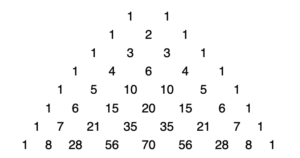# Tossing Coins in Pascal’s TriangleThis array of numbers shows 8 rows of Pascal’s Triangle (it does continue). An interesting relationship exists between the numbers in Pascal’s Triangle and the probability of various outcomes when flipping coins. Investigate this by studying the possible outcomes and associated probabilities of getting number of heads for various numbers of coins.

See if you can discover how you can use Pascal’s Triangle to find the probability of getting exactly 3 heads when six fair coins are tossed? Explain how you can use Pascal’s Triangle to find the probability of getting at least four heads when tossing seven coins.